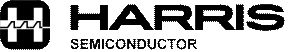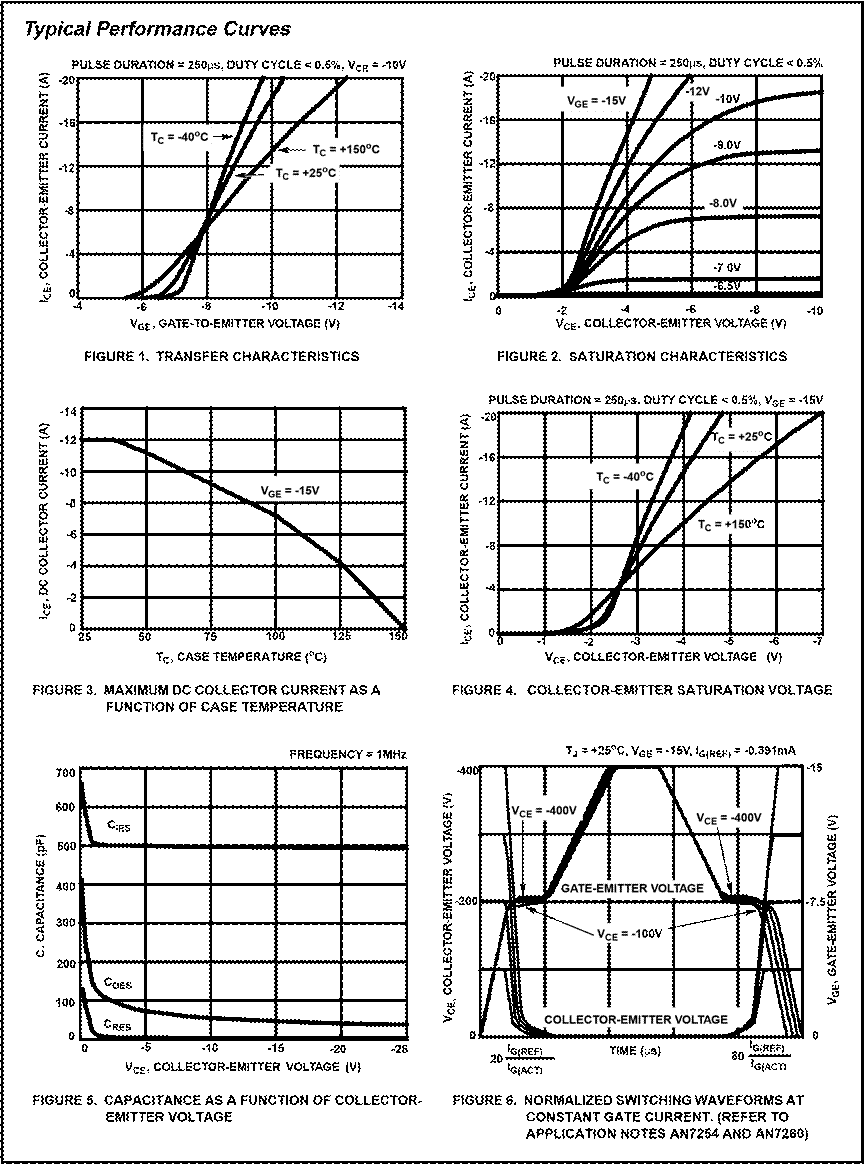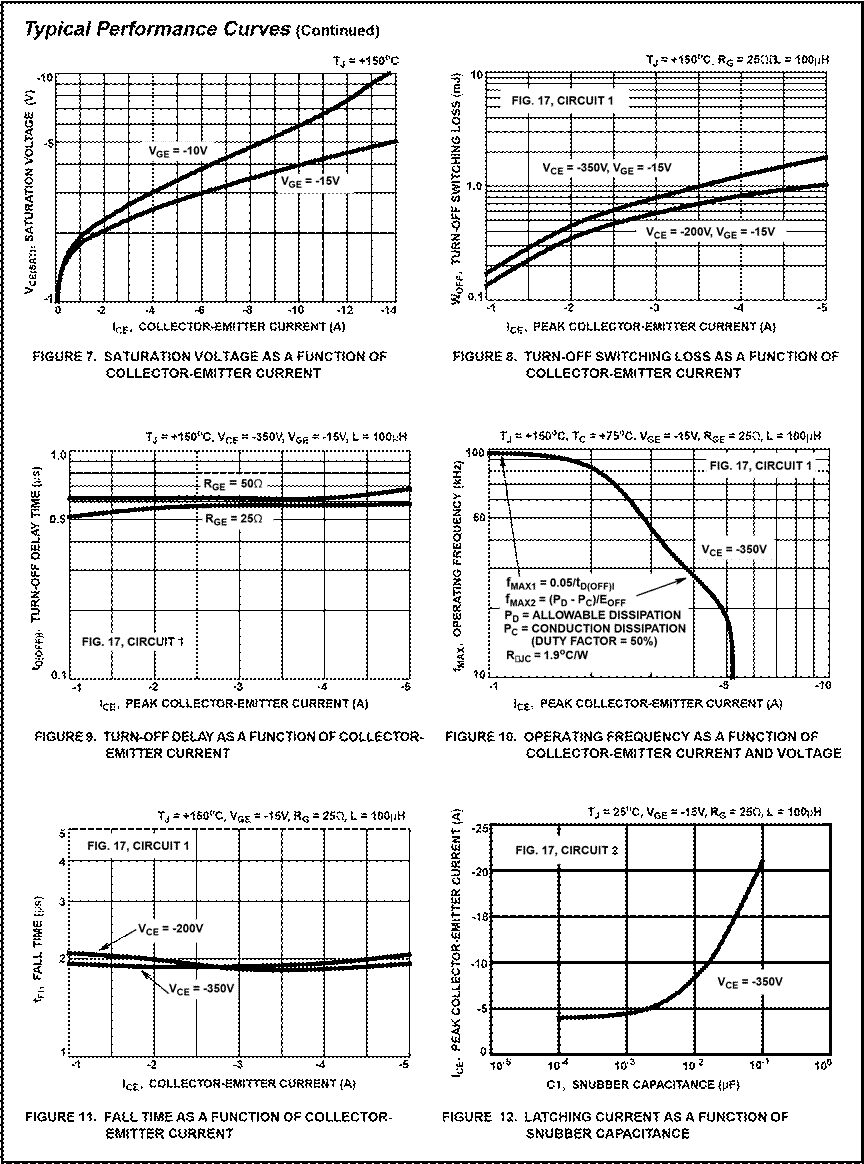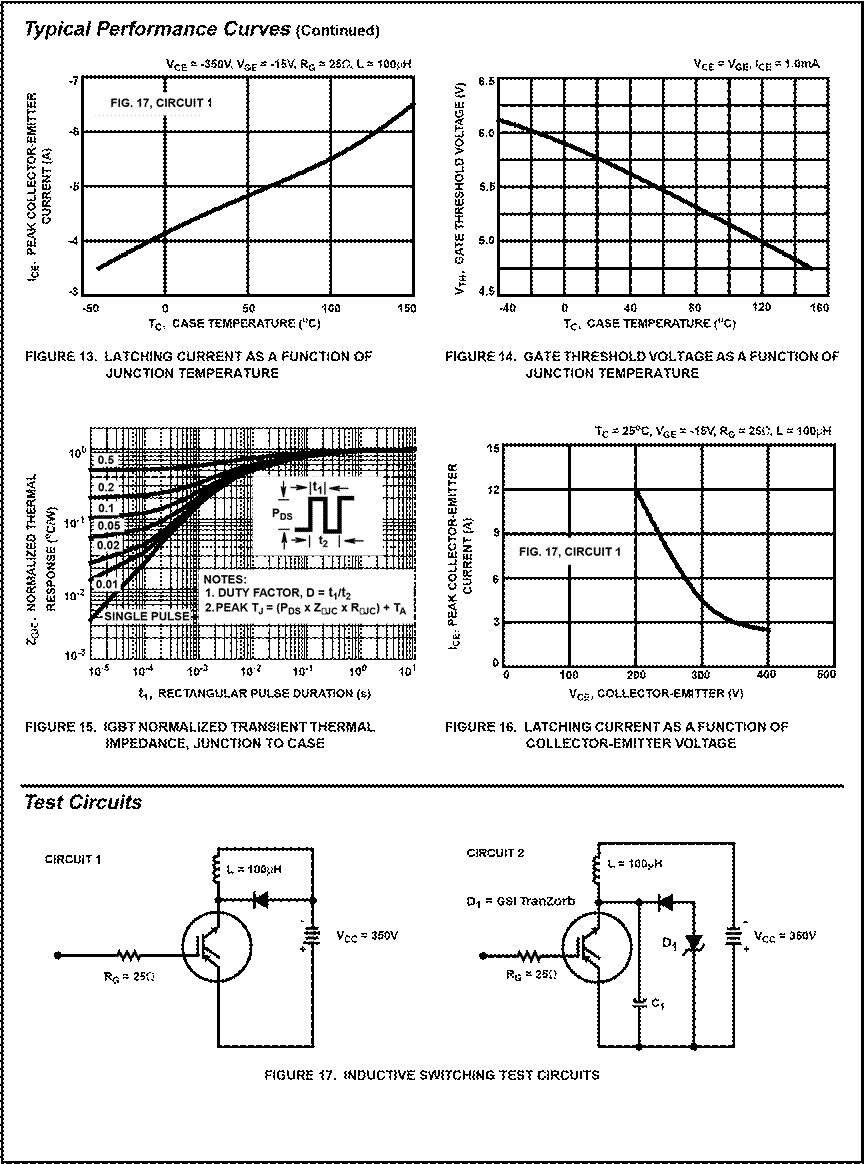# Specifications HGTD8P50G1, HGTD8P50G1S. Electrical Specifications. Collector-Emitter Breakdown Voltage

### Фрагмент текста работыHGTD8P50G1,

HGTD8P50G1S

May 1996                                                                                       8A, 500V P-Channel IGBTsCAUTION: These devices are sensitive to electrostatic discharge. Users should follow proper ESD Handling Procedures.  File Number 3649.2

Specifications HGTD8P50G1, HGTD8P50G1S

Electrical Specifications   TC = +25oC, Unless Otherwise Specified

 PARAMETERS SYMBOL TEST CONDITIONS MIN TYP MAX UNIT Collector-Emitter Breakdown Voltage BVCES ICE = -250µA VCL = -600V VGE = 0V -500 - - V Emitter-Collector Breakdown Voltage BVECS IEC = 1mA VGE = 0V 10 - - V Collector-Emitter Leakage Current ICES VCE = BVCES TC = +25oC - - -250 µA VCE = 0.8 BVCES TC = +150oC - - -1.0 mA Collector-Emitter Saturation Voltage VCE(SAT) ICE = -3.0A VGE = -15V TC = +25oC - -2.5 -2.9 V TC = +150oC - -2.3 -2.8 V ICE = IC90 VGE = -15V TC = +25oC - -3.0 -3.7 V TC = +150oC - -3.3 -4.0 V Gate-Emitter Threshold Voltage VGE(TH) ICE = -1.0mA VCE = VGE -4.5 -6.0 -7.5 V Gate-Emitter Leakage Current IGES VGE = ±20V - - ±100 nA Gate-Emitter Plateau Voltage VGE(PL) IC = 3A VCE = 0.5 BVCES - -7.0 - V On-State Gate Charge QG(ON) IC = 3A, VCE = 0.5 BVCES VGE = -15V - 16 25 nC VGE = -20V - 22 30 nC Current Turn-On Delay Time tD(ON)I RL = 113Ω ICE = -3A, VGE = -15V VCE = -350V RG = 25Ω TJ = +150oC Fig. 17, Circuit 1 - 45 - ns Current Rise Time tRI - 85 - ns Current Turn-off Delay Time tD(OFF)I L = 100µH - 480 680 ns Current Fall Time tFI - 1800 2500 ns Turn-Off Energy (Note 1) EOFF - 0.8 - mJ Current Turn-Off Delay Time tD(OFF)I L = 100µH ICE = -8A, VGE = -15V VCE = -350V RG = 25Ω TJ = +150oC Fig. 17, Circuit 2 C1 = .022µF - 100 200 ns Current Fall Time tFI - 3500 4000 ns Turn-Off Energy (Note 1) EOFF - 1.3 - mJ Latching Current IL L = 100µH VGE = -15V RG = 25Ω TJ = +25oC VCE = -350V Fig. 17, Circuit 1 -3 - - A Thermal Resistance RθJC - 1.75 1.90 oC/W

NOTE:

1. Turn-Off Energy Loss (EOFF) is defined as the integral of the instantaneous power loss starting at the trailing edge of the input pulse and ending at the point where the collector current equals zero (ICE = 0A). The HGTD8P50G1 and HGTD8P50G1S were tested per JEDEC standard No. 24-1 Method for Measurement of Power Device Turn-Off Switching Loss. This test method produces the true total Turn-Off Energy Loss. Turn-On losses include diode losses.Operating Frequency Information              Handling Precautions for IGBTs Operating frequency information for a typical device (Figure Insulated Gate Bipolar Transistors are susceptible to gate10) is presented as a guide for estimating device performance insulation damage by the electrostatic discharge of energy for a specific application. Other typical frequency vs collector through the devices. When handling these devices, care current (ICE) plots are possible using the information shown should be exercised to assure that the static charge built in for a typical unit in Figure 7, Figure 8 and Figure 9. The oper- the handler’s body capacitance is not discharged through ating frequency plot (Figure 10) of a typical device shows the device. With proper handling and application procedures, fMAX1 or fMAX2 whichever is smaller at each point. The infor- however, IGBTs are currently being extensively used in mation is based on measurements of a typical device and is production by numerous equipment manufacturers in bounded by the maximum rated junction temperature. military, industrial and consumer applications, with virtually no damage problems due to electrostatic discharge. IGBTs fMAX1 is defined by fMAX1 = 0.05/tD(OFF)I. tD(OFF)I deadtime can be handled safely if the following basic precautions are (the denominator) has been arbitrarily held to 10% of the ontaken: state time for a 50% duty factor. Other definitions are possible. tD(OFF)I is defined as the time between the 90% 1. Prior to assembly into a circuit, all leads should be kept point of the trailing edge of the input pulse and the point shorted together either by the use of metal shorting where the collector current falls to 90% of its maximum springs or by the insertion into conductive material such value. Device Turn-Off delay can establish an additional fre- as “†ECCOSORBD LD26” or equivalent. quency limiting condition for an application other than TJMAX. 2. When devices are removed by hand from their carriers, tD(OFF)I is important when controlling output ripple under a the hand being used should be grounded by any suitable lightly loaded condition. fMAX2 is defined by fMAX2 = (PD - means - for example, with a metallic wristband. PC)/EOFF. The allowable dissipation (PD) is defined by PD =       3. Tips of soldering irons should be grounded. (TJMAX - TC)/RθJC. The sum of device switching and conduc- 4. Devices should never be inserted into or removed from tion losses must not exceed Pd. A 50% duty factor was used circuits with power on. (Figure 10) and the conduction losses (Pc) are approximated by Pc = (VCE • ICE)/2. EOFF is defined as the integral of the 5. Gate Voltage Rating - Never exceed the gate-voltage instantaneous power loss starting at the trailing edge of the rating of VGEM. Exceeding the rated VGE can result in perinput pulse and ending at the point where the collector manent damage to the oxide layer in the gate region. current equals zero (ICE = 0A).                                                            6. Gate Termination - The gates of these devices are The switching power loss (Figure 10) is defined as fMAX2 • essentially capacitors. Circuits that leave the gate open-circuited or floating should be avoided. These conditions EOFF. Turn-On switching losses are not included because can result in Turn

Предмет:
КР приемники
Тип:
Курсовые работы
Размер файла:
153 Kb
Скачали:
0